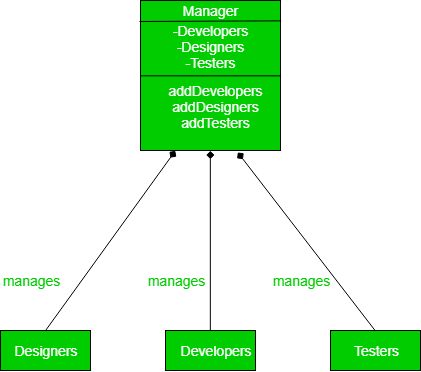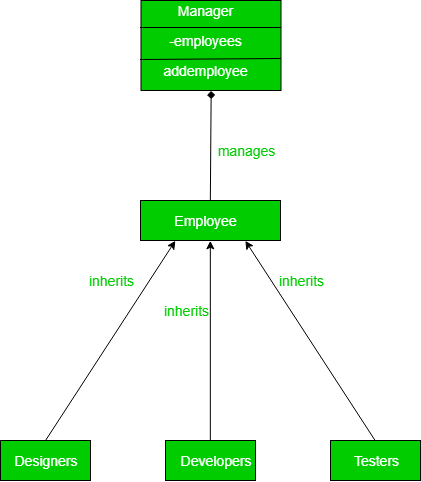Related Articles
Dependency Inversion Principle (SOLID)
• Difficulty Level : Medium
• Last Updated : 27 Mar, 2018

Let’s understand one of the key principles of SOLID principles group namely, Dependency inversion principle.

Dependency inversion principle is one of the principles on which most of the design patterns are build upon. Dependency inversion talks about the coupling between the different classes or modules. It focuses on the approach where the higher classes are not dependent on the lower classes instead depend upon the abstraction of the lower classes. The main motto of the dependency inversion is Any higher classes should always depend upon the abstraction of the class rather than the detail.

This aims to reduce the coupling between the classes is achieved by introducing abstraction between the layer, thus doesn’t care about the real implementation.

Let’s understand the dependency inversion principle with an example. Say you are a manager having some persons as an employee of which some are developers and some are graphic designers and rest are testers.

Now let’s see how a naive design would look without any dependency inversion and what are the loopholes in that design.

 `class` `Manager(``object``):``    ``def` `__init__(``self``):``        ``self``.developers``=``[]``        ``self``.designers``=``[]``        ``self``.testers``=``[]`` ` `    ``def` `addDeveloper(``self``,dev):``        ``self``.developers.append(dev)``         ` `    ``def` `addDesigners(``self``,design):``        ``self``.designers.append(design)``         ` `    ``def` `addTesters(``self``,testers):``        ``self``.testers.append(testers)`` ` `     ` `class` `Developer(``object``):``    ``def` `__init__(``self``):``        ``print` `"developer added"``     ` `class` `Designer(``object``):``    ``def` `__init__(``self``):``        ``print` `"designer added"``     ` `class` `Testers(``object``):``    ``def` `__init__(``self``):``        ``print` `"tester added"``     ` `if` `__name__ ``=``=` `"__main__"``:``    ``a``=``Manager()``    ``a.addDeveloper(Developer())``    ``a.addDesigners(Designer())`
```Output :
developer added
designer added

```

This can be easily be visualized by the following UML Diagram.Now, let’s look at the design loop holes in the source code :
First, you have exposed everything about the lower layer to the upper layer, thus abstraction is not mentioned. That means Manager must already know about the type of the workers that he can supervise.

Now if another type of worker comes under the manager lets say, QA (quality assurance), then the whole class needs to be rejigged. This is where dependency inversion principle pitch in.

Let’s see how to solve the problem to the better extent using Dependency Inversion Principle.

 `  ` `class` `Employee(``object``):``    ``def` `Work():``        ``pass``     ` `class` `Manager():``    ``def` `__init__(``self``):``        ``self``.employees``=``[]``    ``def` `addEmployee(``self``,a):``        ``self``.employees.append(a)``  ` `class` `Developer(Employee):``    ``def` `__init__(``self``):``        ``print` `"developer added"``    ``def` `Work():``        ``print` `"turning coffee into code"``         ` `class` `Designer(Employee):``    ``def` `__init__(``self``):``        ``print` `"designer added"``    ``def` `Work():``        ``print` `"turning lines to wireframes"``         ` `class` `Testers(Employee):``    ``def` `__init__(``self``):``        ``print` `"tester added"``    ``def` `Work():``        ``print` `"testing everything out there"``         ` `if` `__name__ ``=``=` `"__main__"``:``    ``a``=``Manager()``    ``a.addEmployee(Developer())``    ``a.addEmployee(Designer())`
```Output :
developer added
designer added

```

Now if any other kind of the employee is added it can be simply be added to Manager without making the manager explicitly aware of it. Now to add another class of employee we can simply call

```class QA(Employee):
def Work():
print "testing everything out there"
a.add(QA())
```

The creation of the abstraction between different employees and Manager has resulted in very good looking design code which is easily maintainable and extendable.Please have a look into the UML diagram below.In this code, the manager doesn’t have an idea beforehand about all the type of workers that may come under him/her making the code truly decoupled. There are many design patterns where this is a core idea and other things are built upon it.My Personal Notes arrow_drop_up